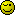# Quick and dumb question

Hello, another brain freeze, I got this code:

``````foreach (\$entryArray as \$key => \$value)
{
\$fields .= "\$key = :\$key, ";
}
\$fields = substr(rtrim(\$fields), 0, -1);
``````

it produces something like “title = :title, duration = :duration,”

The foreach loop introduces a comma at the end of the string which I don’t want or it confuses Mysql, so I used rtrim to get rid of it.

Is there a better way to do it? That is to obtain a comma-separated string with the above pattern from an array and not include the last comma?

Thanks

There is no point in this context. Anthony likes to do things a certain way.

Er, no you didn’t.It might be worthwhile taking a look at using an iterator to ‘decorate’ the data for you, rather than manipulate it.

``````
<?php
class EntryIterator extends ArrayIterator
{
public function key(){
return ':' . parent::key();
}
}

\$entries = array(
'one'   => 1,
'two'   => 2,
'three' => 3,
'four'  => 4,
);

foreach(new EntryIterator(\$entries) as \$key => \$value){
echo \$key ' = ' . \$value . "\
" ;
}

/*
:one = 1
:two = 2
:three = 3
:four = 4
*/
?>

``````

Anyway, I’m glad you got it sorted.damn, I can be very stupid at times, thanks for helping me out of my stupidityAgreed, in this context.I promise I did it, but not in the code I posted, I did it before posting the code so you didn’t see itOn to the iterator then, what is the difference between using an Iterator as you’ve described and creating a new array out of the existing one? Doesn’t the iterator end up doing that anyway? I mean if I changed the echo bit for a declaration of a new array which is what I need to pass to the PDO prepare statement.

You nearly had it.``````
<?php

\$entry = array(
'one'   => 1,
'two'   => 2,
'three' => 3,
'four'  => 4
);

foreach(array_keys(\$entry) as \$key){
\$string .= "{\$key} =:{\$key}, ";
}

echo rtrim(\$string, ', ');
#one =:one, two =:two, three =:three, four =:four

?>

``````

That’s because you did not include the 2nd parameter, the character mask.Funny enough, I tried this first and rtrim did NOT do the trick for some reasonTry this:

``````
\$paramsArray = array();
foreach (\$entryArray as \$key => \$value) {
\$paramsArray[] = "\$key = :\$key";
}
\$fields = implode(', ', \$paramsArray);

``````

\$data2update[“:\$key”] = \$value;

yes I did, I wrote it exactly the way you have it there.

But Immerse’s code did work so thanks a lot.

Now I have another issue with this, I need to build an array with the following structure from these same values:

‘:\$key_name’=> \$value

This of course does not work:

``````\$data2update[':\$key'] = \$value;
``````

Basically I need to build a new array that has the same key names but each preceded with a colon before the name, so

‘title’=> ‘Midnight Run’;
would become
‘:title’=> ‘Midnight Run’;

can’t think of the way to do it! Arrghh, I hate it when I get stupid